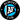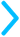# Negative Numbers

We're not huge fans of negative numbers ourselves (they're literally called NEGATIVE numbers 😓), but they're all over the SAT, so we must master them.

#### Are negative numbers even or odd?

They can be both! Just like positive numbers, any negative number divisible by 2 is even, and any odd number not divisible by 2 is odd.

#### Common Questions

They can be both! Just like positive numbers, any negative number divisible by 2 is even, and any odd number not divisible by 2 is odd.

$-1-3 = ?$

Just imagine you're on a number line starting at -1

If we're subtracting, no matter where we start, we're going to move LEFT. Try dragging the 😬 three places to the left.

😬